# Functors, Applicatives, And Monads In Pictures

updated: May 20, 2013

Here's a simple value: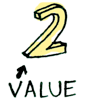And we know how to apply a function to this value:Simple enough. Lets extend this by saying that any value can be in a context. For now you can think of a context as a box that you can put a value in: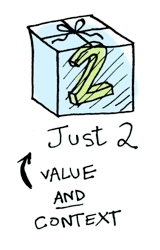Now when you apply a function to this value, you'll get different results depending on the context. This is the idea that Functors, Applicatives, Monads, Arrows etc are all based on. The `Maybe` data type defines two related contexts: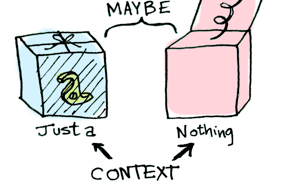``````data Maybe a = Nothing | Just a
``````

In a second we'll see how function application is different when something is a `Just a` versus a `Nothing`. First let's talk about Functors!

## Functors

When a value is wrapped in a context, you can't apply a normal function to it:This is where `fmap` comes in. `fmap` is from the street, `fmap` is hip to contexts. `fmap` knows how to apply functions to values that are wrapped in a context. For example, suppose you want to apply `(+3)` to `Just 2`. Use `fmap`:

``````> fmap (+3) (Just 2)
Just 5
``````Bam! `fmap` shows us how it's done! But how does `fmap` know how to apply the function?

## Just what is a Functor, really?

`Functor` is a typeclass. Here's the definition:A `Functor` is any data type that defines how `fmap` applies to it. Here's how `fmap` works:So we can do this:

``````> fmap (+3) (Just 2)
Just 5
``````

And `fmap` magically applies this function, because `Maybe` is a Functor. It specifies how `fmap` applies to `Just`s and `Nothing`s:

``````instance Functor Maybe where
fmap func (Just val) = Just (func val)
fmap func Nothing = Nothing
``````

Here's what is happening behind the scenes when we write `fmap (+3) (Just 2)`: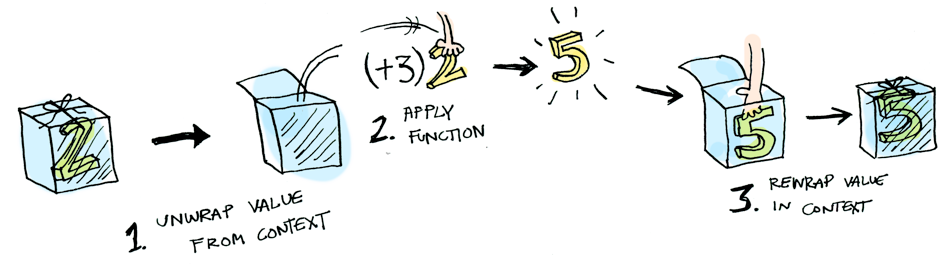So then you're like, alright `fmap`, please apply `(+3)` to a `Nothing`?``````> fmap (+3) Nothing
Nothing
``````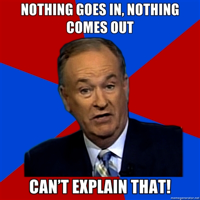Like Morpheus in the Matrix, `fmap` knows just what to do; you start with `Nothing`, and you end up with `Nothing`! `fmap` is zen. Now it makes sense why the `Maybe` data type exists. For example, here's how you work with a database record in a language without `Maybe`:

``````post = Post.find_by_id(1)
if post
return post.title
else
return nil
end
``````

``````fmap (getPostTitle) (findPost 1)
``````

If `findPost` returns a post, we will get the title with `getPostTitle`. If it returns `Nothing`, we will return `Nothing`! Pretty neat, huh? `<\$>` is the infix version of `fmap`, so you will often see this instead:

``````getPostTitle <\$> (findPost 1)
``````

Here's another example: what happens when you apply a function to a list?Lists are functors too! Here's the definition:

``````instance Functor [] where
fmap = map
``````

Okay, okay, one last example: what happens when you apply a function to another function?

``````fmap (+3) (+1)
``````

Here's a function: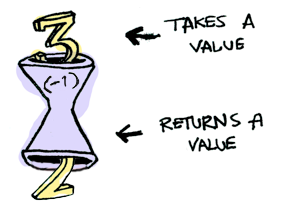Here's a function applied to another function: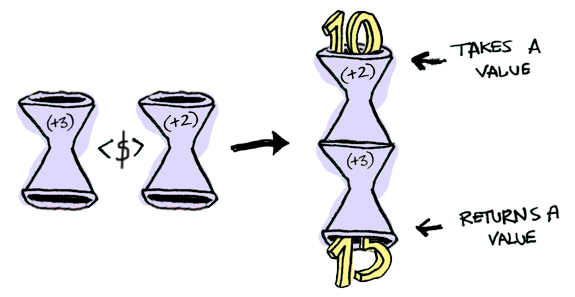The result is just another function!

``````> import Control.Applicative
> let foo = fmap (+3) (+2)
> foo 10
15
``````

So functions are Functors too!

``````instance Functor ((->) r) where
fmap f g = f . g
``````

When you use fmap on a function, you're just doing function composition!

## Applicatives

Applicatives take it to the next level. With an applicative, our values are wrapped in a context, just like Functors:But our functions are wrapped in a context too!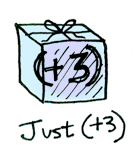Yeah. Let that sink in. Applicatives don't kid around. `Control.Applicative` defines `<*>`, which knows how to apply a function wrapped in a context to a value wrapped in a context: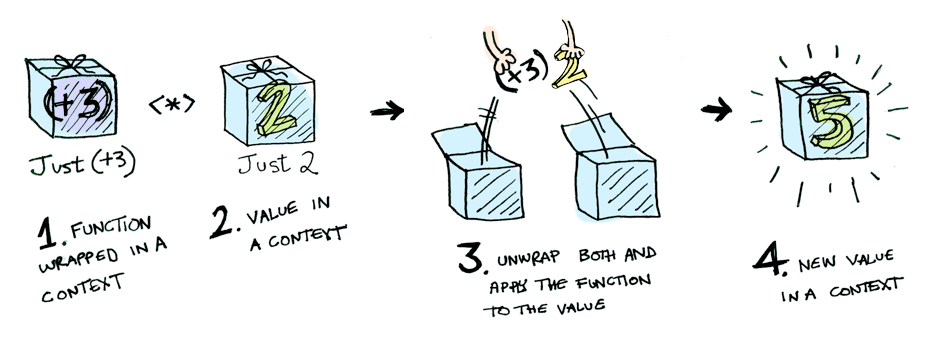i.e:

``````Just (+3) <*> Just 2 == Just 5
``````

Using `<*>` can lead to some interesting situations. For example:

``````> [(*2), (+3)] <*> [1, 2, 3]
[2, 4, 6, 4, 5, 6]
``````Here's something you can do with Applicatives that you can't do with Functors. How do you apply a function that takes two arguments to two wrapped values?

``````> (+) <\$> (Just 5)
Just (+5)
> Just (+5) <\$> (Just 4)
ERROR ??? WHAT DOES THIS EVEN MEAN WHY IS THE FUNCTION WRAPPED IN A JUST
``````

Applicatives:

``````> (+) <\$> (Just 5)
Just (+5)
> Just (+5) <*> (Just 3)
Just 8
``````

`Applicative` pushes `Functor` aside. "Big boys can use functions with any number of arguments," it says. "Armed `<\$>` and `<*>`, I can take any function that expects any number of unwrapped values. Then I pass it all wrapped values, and I get a wrapped value out! AHAHAHAHAH!"

``````> (*) <\$> Just 5 <*> Just 3
Just 15
``````

And hey! There's a function called `liftA2` that does the same thing:

``````> liftA2 (*) (Just 5) (Just 3)
Just 15
``````

1. Get a PhD in computer science.
2. Throw it away because you don't need it for this section!

Functors apply a function to a wrapped value:Applicatives apply a wrapped function to a wrapped value:Monads apply a function that returns a wrapped value to a wrapped value. Monads have a function `>>=` (pronounced "bind") to do this.

Let's see an example. Good ol' `Maybe` is a monad:Suppose `half` is a function that only works on even numbers:

``````half x = if even x
then Just (x `div` 2)
else Nothing
``````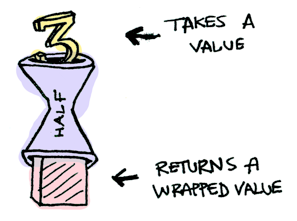What if we feed it a wrapped value?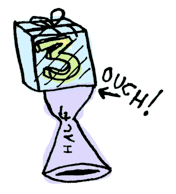We need to use `>>=` to shove our wrapped value into the function. Here's a photo of `>>=`:Here's how it works:

``````> Just 3 >>= half
Nothing
> Just 4 >>= half
Just 2
> Nothing >>= half
Nothing
``````

What's happening inside? `Monad` is another typeclass. Here's a partial definition:

``````class Monad m where
(>>=) :: m a -> (a -> m b) -> m b
``````

Where `>>=` is: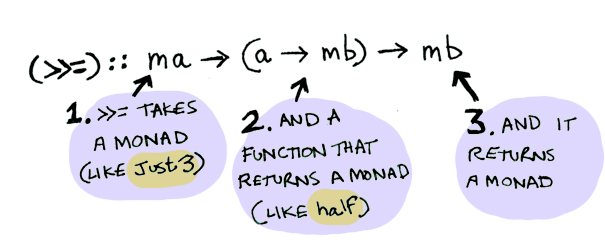So `Maybe` is a Monad:

``````instance Monad Maybe where
Nothing >>= func = Nothing
Just val >>= func  = func val
``````

Here it is in action with a `Just 3`!And if you pass in a `Nothing` it's even simpler: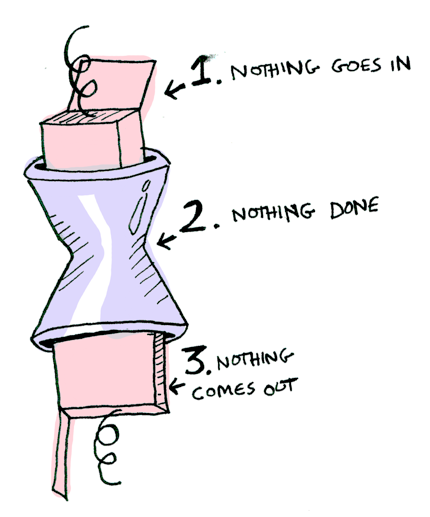You can also chain these calls:

``````> Just 20 >>= half >>= half >>= half
Nothing
``````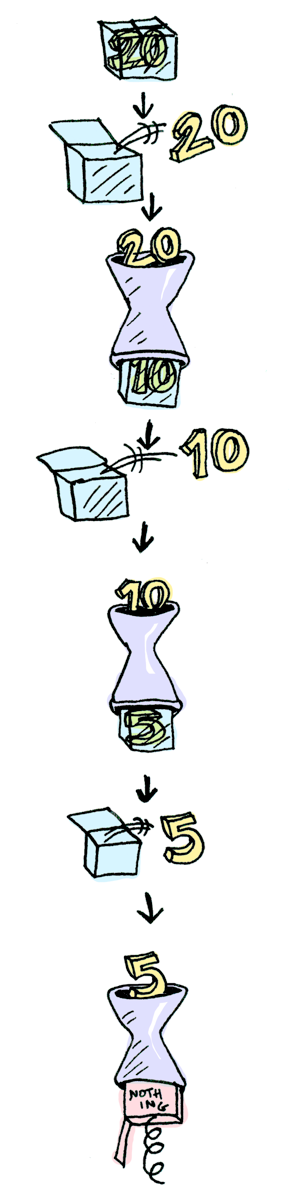Cool stuff! So now we know that `Maybe` is a `Functor`, an `Applicative`, and a `Monad`.

Now let's mosey on over to another example: the `IO` monad:Specifically three functions. `getLine` takes no arguments and gets user input:``````getLine :: IO String
``````

`readFile` takes a string (a filename) and returns that file's contents:``````readFile :: FilePath -> IO String
``````

`putStrLn` takes a string and prints it:``````putStrLn :: String -> IO ()
``````

All three functions take a regular value (or no value) and return a wrapped value. We can chain all of these using `>>=`!``````getLine >>= readFile >>= putStrLn
``````

Aw yeah! Front row seats to the monad show!

Haskell also provides us with some syntactical sugar for monads, called `do` notation:

``````foo = do
filename <- getLine
putStrLn contents
``````

## Conclusion

1. A functor is a data type that implements the `Functor` typeclass.
2. An applicative is a data type that implements the `Applicative` typeclass.
3. A monad is a data type that implements the `Monad` typeclass.
4. A `Maybe` implements all three, so it is a functor, an applicative, and a monad.

What is the difference between the three?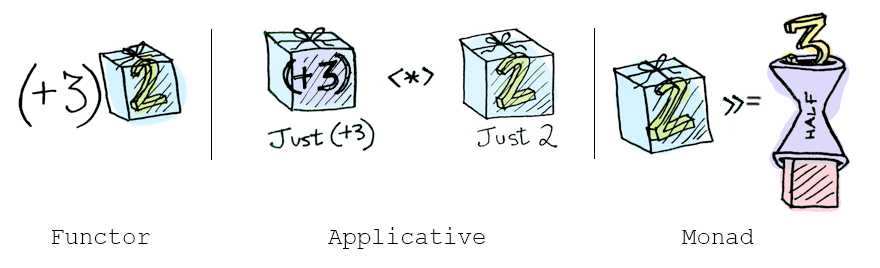• functors: you apply a function to a wrapped value using `fmap` or `<\$>`
• applicatives: you apply a wrapped function to a wrapped value using `<*>` or `liftA`
• monads: you apply a function that returns a wrapped value, to a wrapped value using `>>=` or `liftM`

So, dear friend (I think we are friends by this point), I think we both agree that monads are easy and a SMART IDEA(tm). Now that you've wet your whistle on this guide, why not pull a Mel Gibson and grab the whole bottle. Check out LYAH's section on Monads. There's a lot of things I've glossed over because Miran does a great job going in-depth with this stuff.

## Translations

This post has been translated into:

Human languages:

Programming languages:

If you translate this post, send me an email and I'll add it to this list!